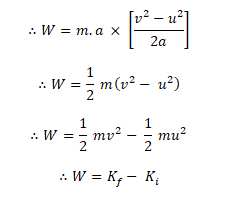FREE ASSISTANCE FOR THE INQUISITIVE PEOPLE
Tutorial Topics
Potential Energy - Fundamentals Different forms of Energy
Kinetic Energy - Fundamentals - Work, Power and Energy
2207    Arnab De    16/10/2018The energy possessed by a body by virtue of its state of motion is called the kinetic energy.

### Expression of kinetic energy

The kinetic energy possessed by a moving body is equal to the amount of work which the moving body performs before coming to rest. It can be calculated by applying an opposing force to stop the body and calculating the amount of work done by the opposing force.

Let, a body of mass m is moving with a velocity v. It is brought to rest by applying a constant opposing force F. Let, a, be the uniform retardation produced by the force and body travels a distance S before coming to rest. Then,

Retarding force F = m a

∴ Kinetic energy of the body = work done by the retarding force in stopping it.

∴ Kinetic energy K = Retarding force × displacement

∴ K = F × S

Now we calculate the displacement S, we have

Initial velocity (u) = v

Final velocity (v) = 0

Since a is the retardation, so acceleration = -a

∴ V2 = u2 +2 a S

∴ 0 = v2 – 2 a S

∴ Displacement S = v2 / 2a

∴ Kinetic energy K = ma (×) (v2 / 2a) = ½ mv2### Work Energy theorem

According the work-energy theorem, the work done by a force on a moving body is equal to the increase in its kinetic energy.

Let a body of mass m be moving with an initial velocity u. When a constant force F is applied on the body along its direction of motion, it produces acceleration a and the velocity of the body change from u to v in moving a distance S,

Force F = mass × acceleration = m . a

∴ W = F X S

Here, S = (v2 – u2) / 2a

W = m a × ((v2 – u2) / 2a)Thus we can say, work done on the body = Increase in kinetic energy

Potential Energy - Fundamentals Different forms of Energy
Author DetailsArnab De
I have over 16 years of experience working as an IT professional, ranging from teaching at my own institute to being a computer faculty at different leading institute across Kolkata. I also work as a web developer and designer, having worked for renowned companies and brand. Through tutorialathome, I wish to share my years of knowledge with the readers.
Enter New Comment
Comment History
No Comment Found Yet.The world is needed a mixture of truth and make-believe. Discard the make-believe and take the truth.
Sri Sri Ramakrishna Paramahamsa
2412
34.21
Today So Far
Total View (Lakh)